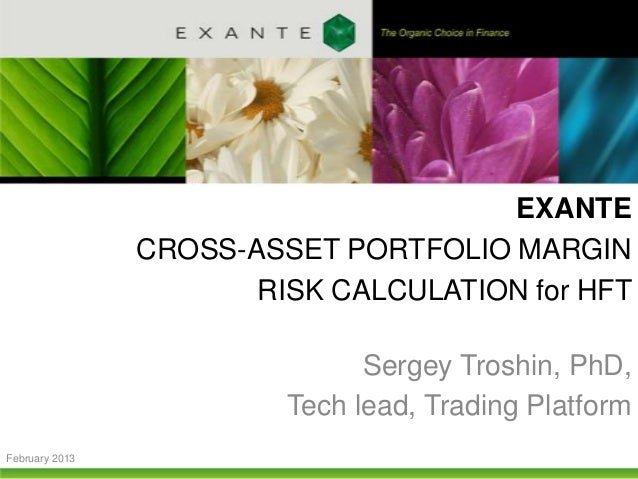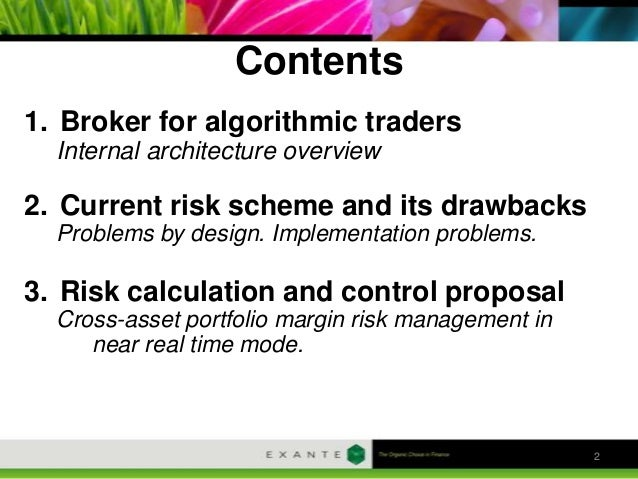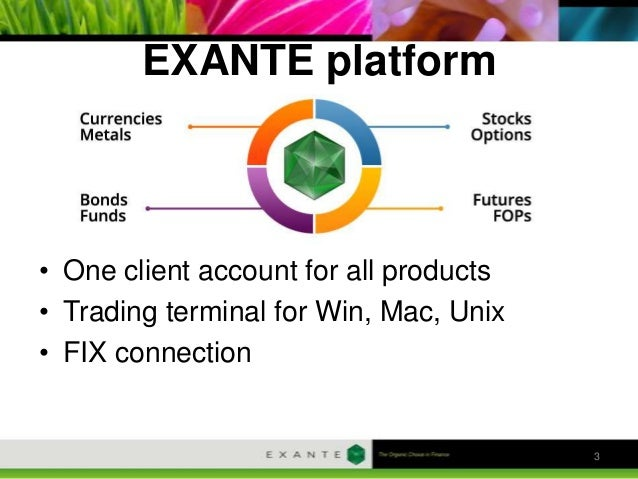Successfully reported this slideshow.Upcoming SlideShare
×

ofUpcoming SlideShare
Extent 2013 Obninsk Test Tools for Trading Systems: Evolution Theory
Next

0 Likes

Share

# Extent 2013 Obninsk Cross-Asset Portfolio Margin Risk Calculation for HFT

EXANTE - "Cross-Asset Portfolio Margin Risk Calculation for HFT"

See all

### Related Audiobooks

#### Free with a 30 day trial from Scribd

See all
• Be the first to like this

### Extent 2013 Obninsk Cross-Asset Portfolio Margin Risk Calculation for HFT

1. 1. EXANTE CROSS-ASSET PORTFOLIO MARGIN RISK CALCULATION for HFT Sergey Troshin, PhD, Tech lead, Trading Platform February 2013
2. 2. Contents 1. Broker for algorithmic traders Internal architecture overview 2. Current risk scheme and its drawbacks Problems by design. Implementation problems. 3. Risk calculation and control proposal Cross-asset portfolio margin risk management in near real time mode. 2
3. 3. EXANTE platform • One client account for all products • Trading terminal for Win, Mac, Unix • FIX connection 3
4. 4. Presence in all major financial centres • 50+ Exchanges • 100K+ Instruments 4
5. 5. • 10+ data vendor gateways • 10+ execution gateways 5
6. 6. Parallel trading via many Gateways using one Account => Accurate risk control is needed 6
7. 7. Current Risks 7
8. 8. Current Risks Initial startup After each trade 8
9. 9. Current Risks Pros: • Simple and extremely fast Gateway Cons: • Limits update are not fast enough because of heavy calculations on Back Office side • Orders data are encapsulated in Gateway • Portfolio Margin is not calculated • High BO=>RA traffic (symbols * accounts) • Gateway restart takes significant time • Possible inaccurate limits 9
10. 10. Risks Requirements • 100K symbols and 10K accounts • Fast risk calculation, updates and checks • Use orders data in risk processing • Portfolio margin calculation • Reasonable traffic (symbols + accounts) 10
11. 11. Risks Proposal SymbolLimit = FreeMoney * LeverageRate * CrossCourse / Price Definitions • MarginCoefficient = CrossCourse / Price (initial margin to open position with quantity set to 1 and leverage rate set as 1) Facts • Margin Coefficient doesn’t depend on account • LeverageRate is the same for many accounts • CrossCourse and Price can not change 11
12. 12. Risks Proposal Initial startup Trade processing 12
13. 13. Free Money Account Margin = SUM(Asset Margin) + SUM(Cross-Asset Margin) Asset Margin = Quantity * LeverageRate * MarginCoefficient Cross-Asset Margin = SPAN-like algorithm for same underlying 13
14. 14. Improvements 1. Move free money calculation to Risk Agent. Orders synchronization is needed. 2. Compute free money using cross margining info 3. Use margin data in ATP 14
15. 15. Proposed risks Pros: • Gateway is still very fast(<1ms) • Orders are used in limits calculation • Portfolio Margin can be calculated in different ways • Cross margin data could be used • All limits and leverage rates could be overridden • Fast as light risk updates • Backup gateways Cons: 15
16. 16. www.exante.eu Questions? Sergey Troshin, PhD, Tech Lead (st@exante.eu) 16

Total views

1,817

On Slideshare

0

From embeds

0

Number of embeds

861

0

Shares

0